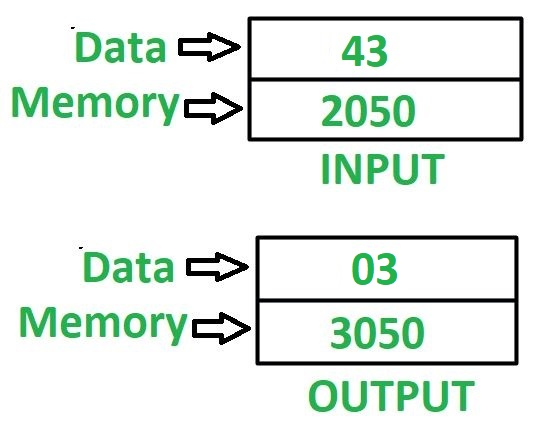GFG App
Open AppBrowser
Continue

# 8085 program to find minimum value of digit in the 8 bit number

Problem – Write an assembly language program in 8085 microprocessor to find minimum value of digit in the 8 bit number. Example – Assume 8 bit number is stored at memory location 2050, and minimum value digit is stored at memory location 3050.Algorithm –

1. Load the content of memory location 2050 in accumulator A.
2. Move the content of A in register B.
3. Perform AND operation of content of A with 0F and store the result in A.
4. Move the content of A in register C.
5. Move the content of B in A.
6. Reverse the content of A by using RLC instruction 4 times.
7. Perform AND operation of content of A with 0F and store the result in A.
8. Compare the contents of A and C by help of CMP C instruction.
9. Check if carry flag is set then jump to memory location 2013 otherwise move the content of C in A. Go to memory location 2013.
10. Store the value of A in memory location 3050.

Note – CMP C instruction compares the value of A and C. If A>C then carry flag becomes reset otherwise set. Program –

Explanation – Registers A, B, C are used for general purpose.

1. LDA 2050: loads the contents of memory location 2050 in A.
2. MOV B, A: moves the content of A in B.
3. ANI 0F: perform AND operation between contents of A and value 0F.
4. MOV C, A: moves the content of A in C.
5. MOV A, B: moves the content of B in A.
6. RLC: shift the content of A left by 1 bit without carry. Use this instruction 4 times to reverse the content of A.
7. ANI 0F: perform AND operation between contents of A and value 0F.
8. CMP C: compare contents of A, C and update the value of carry flag accordingly.
9. JC 2013: jump to memory location 2013 if CY = 1.
10. MOV A, C: moves the content of C in A.
11. STA 3050: stores the content of A in memory location 3050.
12. HLT: stops executing the program and halts any further execution.

1. Simple and easy to understand logic

2. Efficient use of the accumulator and registers

3. Can be easily modified to find the maximum value of a digit